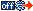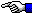NOT to be reproduced in any form without Permission of the Author!

The Decimal number system is also known as Base Ten, since it's comprised of ten numerals (symbolized by 0 through 9). Although we can only represent up to the number 9 by a single decimal digit, it's possible to reference up to ten items by using zero (0) as an index to refer to the first (1st) item; thus, the numeral 9 would refer to the tenth (10th) item. With two digits we can refer to 100 items (zero through 99). In terms of the number of digits, 10n (where n is the number of digits) equals the maximum number of items we can refer to. As we'll see below, this concept is very important in understanding how we use both memory and media to store data on our computers.

In the Binary, or Base 2 number system, there are only two numerals, symbolized by 0 and 1, and its digits are often referred to as bits (from binary digit). Just as ten items can be indexed with a single decimal digit, we can also refer to two items with a single bit; the maximum number being 2n for up to n bits, whereas the largest positive number we could represent using the same number of bits would be: 2n - 1.

Therefore, the decimal equivalent of the largest binary number we can represent in 6 bits (111111) can be found as the sum of the first six powers of 2; starting with 2 to the power of zero (2^0):
20 + 21 + 22 + 23 + 24 + 25 = 1 + 2 + 4 + 8 + 16 + 32 = 63.
Or, by simply using the formula: 2n - 1 = 64 - 1 = 63.

To convert any binary number to hexadecimal, that is, base 16, simply order the bits into as many four-bit groups as possible, from the least significant position to the most significant postition with any remaining group of only 3, 2 or 1 bits at the far left. Then convert each group to a single hex digit of 0 through 9 or A through F. So, to convert our 6-bit number of 111111 (63 decimal) to hex, we simply group the bits as: 11 1111 which is easily converted to: 3F hex. [For more details on the use of Hexadecimal, see: Hexa What?]

An 8-bit or 1-byte hexadecimal number can contain a maximum value of 255 decimal. However, if we begin counting with the number 0 (zero), for example, as the first address where data is stored in memory (or in an LBA sector on a hard disk), we have one more location than normally allowed by the hex number FFh: We have room for 256 addresses. But due to a programming error made long ago (see table below), there are never more than 255 heads in a partition table.

A 10-bit binary number cannot exceed: 11 1111 1111 or 3FFh or 1023 decimal. But, the maximum number of Cylinders we can reference in 10 bits is 1024, since we begin counting from zero.

NOTE: In the Table below, the column labeled "Using Zero" indicates whether or not the number we're counting up to in the "Examples" column begins with zero for the "Maximum Count" we've listed:

 Bits Bytes Words Max. Hex Number Maximum Count Examples UsingZero 6 3F           (63) 63 CHS    Sector Count No 8 1 FF          (255) (See: Note 1)     256 CHS       Head Count Yes 10 3FF       (1023) 1024 CHS Cylinder Count Yes 16 2 1 FFFF (2)               65,535 Hex  Word No 20 F FFFF 1,048,576 (1024 KiB = 1 MiB) Yes 24 3 FF FFFF 16,777,216 (16 MiB) Yes 28 FFF FFFF (3)    268,435,456 BIOS Sector Count Yes 30 3FFF FFFF 1,073,741,824 (1024 MiB = 1 GiB) Yes 32 4 2 FFFF FFFF (4)  4,294,967,295 Hex  Double Word No 40 5 FF FFFF FFFF 1,099,511,627,776 (1024 GiB = 1 TiB) Yes 48 6 3 FFFF FFFF FFFF (Note 5)   (256 TiB) New BIOS Sector Count Yes 50 3 FFFF FFFF FFFF (1024 TiB = 1 PiB) Yes 56 7 FF FFFF FFFF FFFF (64 PiB) Yes 64 8 4 FFFF FFFF FFFF FFFF ( Note 6 ) Hex  Quad Word No

Note 1: Although the maximum number of "Heads" could have been 256 (since this count does begin with zero), there was a programming error in MS-DOS which limited the number of heads to 255 (so we count from 0 through 254). Thus, the limits on the values for CHS tuples inside a partition table are: (1023, 254, 63).

Note 2: The first FAT16 partitions were limited to only 32 MiB in size. This comes from the fact they could handle only 0h through FFFFh ( 65,536 ) sectors. Multiplying that by 512 bytes per sector, gives us: 33,554,432 bytes, or exactly 32 binary MegaBytes.

Note 3: The BIOS code in many now older computers used only 28-bits to count sectors, which limited those machines to handling drives no larger than 137 GB: After multiplying 268,435,456 sectors by 512 bytes per sector, we obtain the limit of: 137,438,953,472 bytes (or exactly 128 GiB -- binary GigaBytes).

Note 4: When partitioning drives larger than about 7.84 GiB or 8.4 GB (1024 x 255 x 63 = 16,450,560 sectors; or exactly: 16450560 x 512 bytes/sector = 8,422,686,720 bytes) using the Master Boot Record scheme, its partitions can still be recorded in one of the same four 16-byte long Partition Table entries that PCs have been using since MS-DOS 2.00 was introduced, because both the Starting Sector and Total Sectors (which are the only values an OS or a utility program uses when a partition extends beyond 1024 cylinders) are stored in 32-bits. This gives them a maximum count of: 4,294,967,296 sectors, for a drive size of almost 2.2 TB (TeraBytes): 2,199,023,255,552 bytes (or exactly 2,048 GiB).

Note 5: Later BIOS chips made use of 48-bits to count the number of LBA blocks a hard disk can store data on. This allows computers to handle drives with 281,474,976,710,656 sectors, for a maximum drive size up to: 144,115,188,075,855,872 bytes (or exactly 134,217,728 GiB, 131,072 TiB and 128 PiB); this last abbreviation stands for: Binary PetaBytes.

Note 6: A 64-bit (or 8-byte) hex number, sometimes called a "Quad Word," has a maximum value of: 18,446,744,073,709,551,615. This is the largest  Hexadecimal number the built-in Windows™ 2000/XP Calculator program can handle(6a). This 64-bit number grows up through Kilo- (10^3), Mega- (10^6), Giga- (10^9), Tera- (10^12), Peta- (10^15) to over 18.4 Exa Bytes (18.4 x 10^18). Or, if we begin counting from zero, exactly 16 Binary Exa Bytes! But that's just getting started if you want to discuss really big numbers! You can read about Zetta- and Yotta- and some bogus names for these and other numerical prefixes, here:Official SI metric prefixes.

____________
6a:
Exact decimal numbers being limited to: 10^32 - 1; a string of thirty-two 9s, after which, values are approximated in scientific notation using a mantissa of up to 32 digits, plus a base 10 exponent (represented by "e+"). This program behaves rather oddly when large exponents are involved. For example, we've been unable to compute a value larger than only 1.e+43429 using the x^y function, yet we've performed multiplication operations with results far in excess of that limit, such as: 1.e+1171773 (a 7-digit exponent). Note: When performing such calculations, Windows may pop-up a box stating it is taking some time to complete, and will ask if you wish to continue.

When using the program's Edit --> Paste function, it's impossible to ENTER a number exceeding only 1.e+9999. If you try to ENTER anything with more than a 4-digit exponent, it's truncated to only the first 4 digits! Yet we had no problem copying much larger results using its Edit --> Copy selection. Other operations, may result in an "Invalid input for function" error message.

Updated: April 13, 2007; August 30, 2009; June 19, 2011; July 24, 2011; May 11, 2012.
Last Update: November 11, 2012.Assembly Language Index PageThe Starman's Realm Index Page